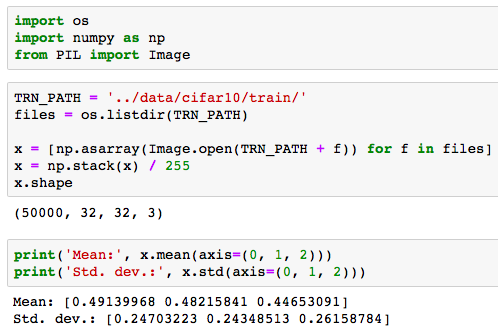# Lec 7 Data stats array

For cifar dataset how was the stats array calculated and what does it represents ? Lec7 notebook

stats = (np.array([ 0.4914 , 0.48216, 0.44653]), np.array([ 0.24703, 0.24349, 0.26159]))It represents the mean and standard deviation for each channel (red, green, blue) on the training set.

2 Likes